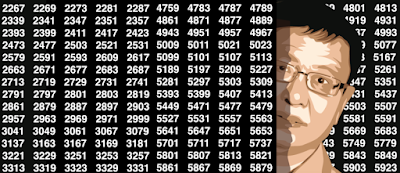## Wednesday, October 2, 2019

### Surprising Uses of Synthetic Random Data Sets

I have used synthetic data sets many times for simulation purposes, most recently in my articles Six degrees of Separations between any two Datasets and How to Lie with p-values. Many applications (including the data sets themselves) can be found in my books Applied Stochastic Processes and New Foundations of Statistical Science. For instance, these data sets can be used to benchmark some statistical tests of hypothesis (the null hypothesis known to be true or false in advance) and to assess the power of such tests or confidence intervals. In other cases, it is used to simulate clusters and test cluster detection / pattern detection algorithms, see here.  I also used such data sets to discover two new deep conjectures in number theory (see here), to design new Fintech models such as bounded Brownian motions, and find new families of statistical distributions (see here).
Goldbach's comet
In this article, I focus on peculiar random data sets to prove -- heuristically -- two of the most famous math conjectures in number theory, related to prime numbers: the Twin Prime conjecture, and the Goldbach conjecture. The methodology is at the intersection of probability theory, experimental math, and probabilistic number theory. It involves working with infinite data sets, dwarfing any data set found in any business context.